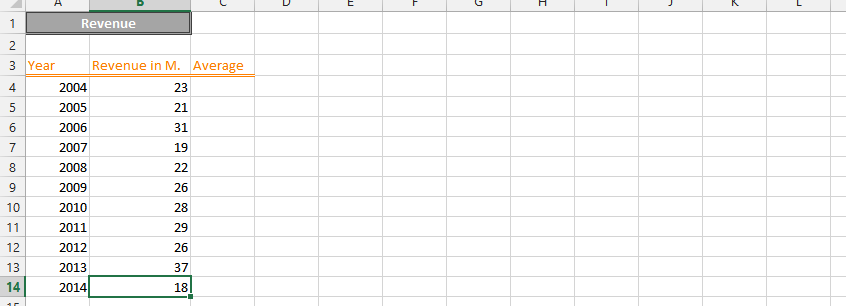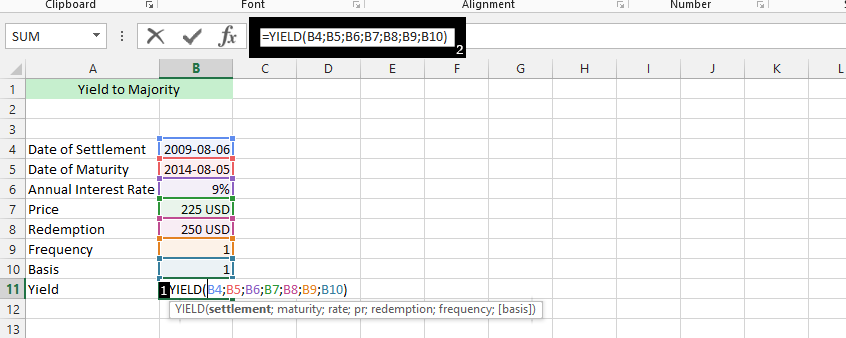#### How to Calculate Yield to Maturity in Excel

Yield to maturity (YTM) is the total return expected from a bond if the investor holds it until maturity, taking into account the current market price, coupon payments, and the face value of the bond.

YTM is academically defined as market interest rate, but means Yield to Maturity. It actually takes purchase price, the value of redemption, time between payment of interest, and the yield of coupon.

## Data preparation

We will calculate the YTM at ease, and you need a data that looks like this:Important Note: It is extremely important that the frequency is 1 – 4, and the basis is 1 – 3. This would guarantee the calculation of the YTM being successful.

## Yield formula creation

Click beside Yield (B11) (1), type =YIELD(B4;B5;B6;B7;B8;B9;B10) (2), and press enter.Note: You can now format the yield to any format you want.

## IRR formula creation

• List the cash flows for the bond, including the coupon payments and the face value of the bond.
• Enter the current market price of the bond.
• Select the cell where you want the Yield to Maturity calculation to appear.

Enter the formula:

• The formula for Yield to Maturity in Excel is: =IRR(values, [guess]).
• Values is the range of cells that contains the cash flows for the bond.
• Guess is an optional argument that provides an initial estimate of the Yield to Maturity.

Press Enter:

• The Yield to Maturity calculation will appear in the selected cell.